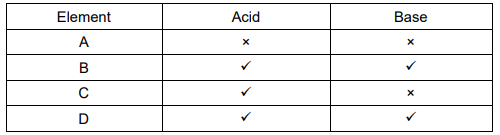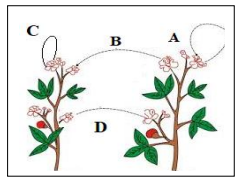# Class 10 Science Board Exam Sample Paper -1

In this page we have Class 10 Science Board Exam Sample Paper -1 . Hope you like them and do not forget to like , social share and comment at the end of the page.

## Section A

Question 1
What is the far point and near point of the human eye with normal vision?

Question 2
Fossils provide evidences for organic evolution.
(a)What are fossils?
(b)What are the different methods for estimating the age of fossils?

Question 3
True and False statement
(a) The spacing between the magnetic lines of forces increases as we move away from current carrying conductor?
(b) The graph between V and I for metals is a curve.
(c) Electric motor converts electrical energy into mechanical energy
(d) Insulators have high resistivity and negligible conductivities.
(f) Current does not gets divided in series combination.
(g)Voltage get divided in parallel combination
(h) Current increases substantially at the time of short circuit
(i) 1 Watt = 1 Volt / 1 Ampere
(j) MRI stands for magnetic resonance imaging

Question 4
What is the site of complete digestion in our body? Name the end products formed?

Question 5
What is observed when a solution of potassium iodide solition is added to a solution of lead nitrate? Name the type of reaction. Write balanced chemical equation?

Question 6
How is amount of urine produced is regulated?

Question 7
Describe:
(a)Lymphatic system
(b) excretion in plants
(c) corrosion
(d) Rancidity
(e) blood pressure

Question 8
(a) What is meant by the term 'frequency' of an alternating current?
(b) What is its value in India?
(c) An A.C has a frequency of 50 Hz. How many times does it change its direction in one second?
(d) Why is an alternating current considered to be advantageous over direct current for long range transmission of electric energy?

Question 9.
What is meant by reflex action? With the help of labelled diagram trace the sequence of events which occur when we touch a hot object.

Question 10
Describe chemotropism with example?

## Section B

Question 11
Give the scientific term for the increase in the amount of non-biodegradable substances in the successive trophic levels of a food chain?

Question 12
Which chemical is used in fire extinguisher? How it is harmful?

Question 13
What is mean by sustainable development?

Question 14
What is meant by DNA copying/ mention its importance?

Question 15
(i)What is the main purpose of rain water harvesting?
(ii)Write two traditional water harvesting  system in India?

Question 16
What are the function performed by testis in human beings?

Question 17
Refractive index of diamond with respect to glass is 1.6 and absolute refractive index of glass is 1.5. Find out the absolute refractive index of diamond.

Question 18
Rays from Sun converge at a point 15 cm in front of a concave mirror. Where should an object be placed so that size of its image is equal to the size of the object?
A. 30 cm in front of the mirror
B. 15 cm in front of the mirror
C. Between 15 cm and 30 cm in front of the mirror
D. More than 30 cm in front of the mirror

Question 19
The table given below shows the reaction of a few elements with acids and bases to evolve Hydrogen gas.Which of these elements form amphoteric oxides?
A. A and D
B. B and D
C. A and C
D. B and D

Question 20
The diagram shown below depicts pollination. Choose the options that will show a maximum variation in the offspring.(a) A, B and C
(b) B and D
(c) B, C and D
(d) A and C

## Summary

This Class 10 Science Board Exam Sample Paper -1 is prepared keeping in mind the latest syllabus of CBSE . This has been designed in a way to improve the academic performance of the students. If you find mistakes , please do provide the feedback on the mail.